# Creating custom steps functions

#### 2022-10-05

Perhaps one of the most useful features of steps is its flexibility. Users have the options of selecting pre-defined functions or writing their own functions to use in simulations. This vignette will describe how to implement custom functions in the steps software.

library(steps)
library(raster)
library(viridisLite)
library(future)

To better facilitate the use of custom functions, a basic understanding of the steps internal structure is required. We have designed a simple and straight-forward application programming interface (API). In its most basic operation, steps passes landscape objects and iterator values (timesteps) to functions. These functions then return landscape objects that have been modified based on operations specified in the functions:

Landscape objects contain information on populations, carrying capacities, habitat features, and other spatially-explicit information used by dynamic functions in a simulation. Dynamics functions act on these landscape objects during a simulation.

Let’s examine a pre-built steps dynamics function “mortality” which changes populations based on spatially-explicit values provided in a raster layer.

mortality <- function (mortality_layer, stages = NULL) {

pop_dynamics <- function (landscape, timestep) {

population_raster <- landscape$population nstages <- raster::nlayers(population_raster) # get population as a matrix idx <- which(!is.na(raster::getValues(population_raster[]))) population_matrix <- raster::extract(population_raster, idx) mortality_prop <- raster::extract(landscape[[mortality_layer]][[timestep]], idx) if (is.null(stages)) stages <- seq_len(nstages) for (stage in stages) { population_matrix[ , stage] <- ceiling(population_matrix[ , stage] * mortality_prop) } # put back in the raster population_raster[idx] <- population_matrix landscape$population <- population_raster

landscape

}

as.population_modification(pop_dynamics)

}

In the first statement, we have defined a function with parameters that specify the name of the layer in the landscape object that will be used to modify populations (mortality_layer) and which life stages will be modified (stages). We have set “stages” to NULL as a default so, if not specified, all stages will be modified. These are global parameters for the function and are optional.

We define an internal function (returned by the main function) in the second statement and this is where the dynamic operations are specified. Note the two parameters are “landscape” and “timestep” and these are both required.

The next few statements operate on the landscape object by extracting the populations of the affected stages, extracting the values of the “mortality layer”, multiplying them to get new populations, and then returning the modified populations to the landscape object. Note the last statement is always “landscape” so the landscape object is returned when the function concludes its operations.

The final statement assigns a class to the function. This is optional but useful to maintain an organised structure in the software. We recommend using the appropriate function based on the category of the function a user is defining. In this case, this function is a “population_modification” class since its operations change the population in the landscape object.

So let’s go about creating a custom function for use in steps. First, we load some initial kangaroo populations:

egk_pop
## class      : RasterStack
## dimensions : 35, 36, 1260, 3  (nrow, ncol, ncell, nlayers)
## resolution : 500, 500  (x, y)
## extent     : 319000, 337000, 5817000, 5834500  (xmin, xmax, ymin, ymax)
## crs        : +proj=utm +zone=55 +south +ellps=GRS80 +towgs84=0,0,0,0,0,0,0 +units=m +no_defs
## min values :        1,        1,     1
## max values :       20,       20,    20
par(mar=c(0,0,0,0), oma=c(0,0,0,0))
spplot(egk_pop,
col.regions = viridis(100),
par.settings=list(strip.background = list(col = "white")))
## Warning in wkt(obj): CRS object has no comment

## Warning in wkt(obj): CRS object has no comment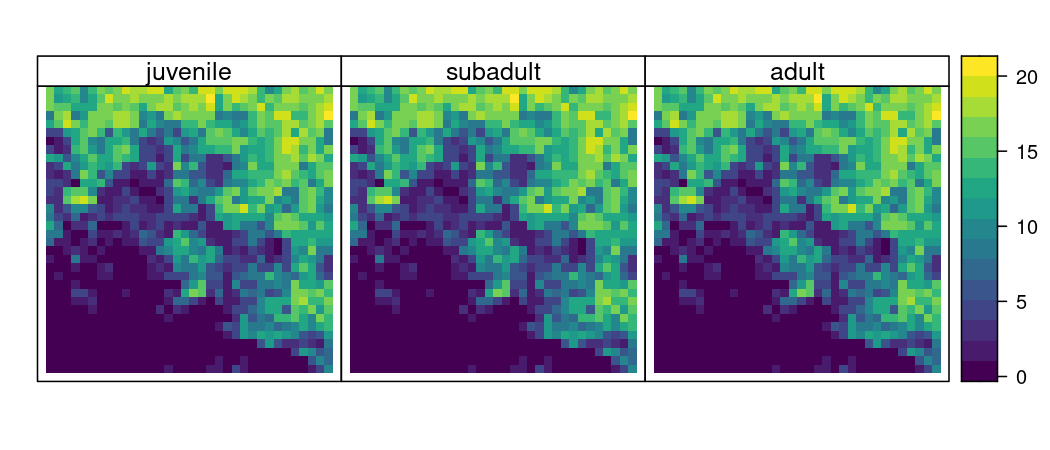Let’s examine the total population of juveniles:

cellStats(egk_pop[], sum)
##  9066

Now, let’s create a function that reduces cell populations randomly across the landscape by a given percentage for the life stages specified. We start with the first two lines coded similar to the previous example and change the function name and one of the parameters:

percent_mortality <- function (percentage, stages = NULL) {

pop_dynamics <- function (landscape, timestep) { ...

Next we extract the populations similar to the previous example. We use an index to avoid extracting missing values:

percent_mortality <- function (percentage, stages = NULL) {

pop_dynamics <- function (landscape, timestep) {
population_raster <- landscape$population nstages <- raster::nlayers(population_raster) # get population as a matrix idx <- which(!is.na(raster::getValues(population_raster[]))) population_matrix <- raster::extract(population_raster, idx) ... We then randomly sample cells (by non-zero indices) to be modified through an iterative process and then stop when we reach our specified target percentage reduction: percent_mortality <- function (percentage, stages = NULL) { pop_dynamics <- function (landscape, timestep) { population_raster <- landscape$population
nstages <- raster::nlayers(population_raster)

# get population as a matrix
idx <- which(!is.na(raster::getValues(population_raster[])))
population_matrix <- raster::extract(population_raster, idx)

if (is.null(stages)) stages <- seq_len(nstages)
for (stage in stages) {
initial_pop <- sum(population_matrix[ , stage])
changing_pop <- sum(population_matrix[ , stage])
while (changing_pop > initial_pop * percentage) {
non_zero <- which(population_matrix[idx , stage] > 0)
i <- sample(non_zero, 1)
population_matrix[i , stage] <- population_matrix[i , stage] - 1
changing_pop <- sum(population_matrix[ , stage])
}
} ...

Finally, similar to the original example, we return the modified populations to the landscape object and then return it from the function:

percent_mortality <- function (percentage, stages = NULL) {

pop_dynamics <- function (landscape, timestep) {
population_raster <- landscape$population nstages <- raster::nlayers(population_raster) # get population as a matrix idx <- which(!is.na(raster::getValues(population_raster[]))) population_matrix <- raster::extract(population_raster, idx) if (is.null(stages)) stages <- seq_len(nstages) for (stage in stages) { initial_pop <- sum(population_matrix[ , stage]) changing_pop <- sum(population_matrix[ , stage]) while (changing_pop > initial_pop * (1 - percentage)) { non_zero <- which(population_matrix[ , stage] > 0) i <- sample(non_zero, 1) population_matrix[i , stage] <- population_matrix[i , stage] - 1 changing_pop <- sum(population_matrix[ , stage]) } } population_raster[idx] <- population_matrix landscape$population <- population_raster
landscape
}
} 

Now that we have a custom function defined in the R environment we can specify it in the model setup. Note, we specify a 10% (percentage = 0.1) reduction in the total landscape population of juveniles (stages = 1) and run the simulation for a single replicate of three iterations (timesteps = 3):

ls <- landscape(population = egk_pop,
suitability = NULL,
carrying_capacity = NULL)

pd <- population_dynamics(change = NULL,
dispersal = NULL,
modification = percent_mortality(percentage = 0.1,
stages = 1),
density_dependence = NULL)

results <- simulation(landscape = ls,
population_dynamics = pd,
habitat_dynamics = NULL,
demo_stochasticity = "none",
timesteps = 3,
replicates = 1,
verbose = FALSE)

We can view the initial total juvenile landscape population and the modified population in each of the timesteps:

cbind(c("t0", "t1", "t2", "t3"),
c(cellStats(egk_pop[], sum),
cellStats(results[][][["population"]][], sum),
cellStats(results[][][["population"]][], sum),
cellStats(results[][][["population"]][], sum)))
##      [,1] [,2]
## [1,] "t0" "9066"
## [2,] "t1" "8159"
## [3,] "t2" "7343"
## [4,] "t3" "6608"

And here is a plot of the temporally-changing juvenile cell populations:

pop_stack <- stack(egk_pop[],
results[][][["population"]][],
results[][][["population"]][],
results[][][["population"]][])

names(pop_stack) <- c("t0", "t1", "t2", "t3")

par(mar=c(0,0,0,0), oma=c(0,0,0,0))
spplot(pop_stack,
col.regions = viridis(100),
par.settings=list(strip.background = list(col = "white")))
## Warning in wkt(obj): CRS object has no comment

## Warning in wkt(obj): CRS object has no comment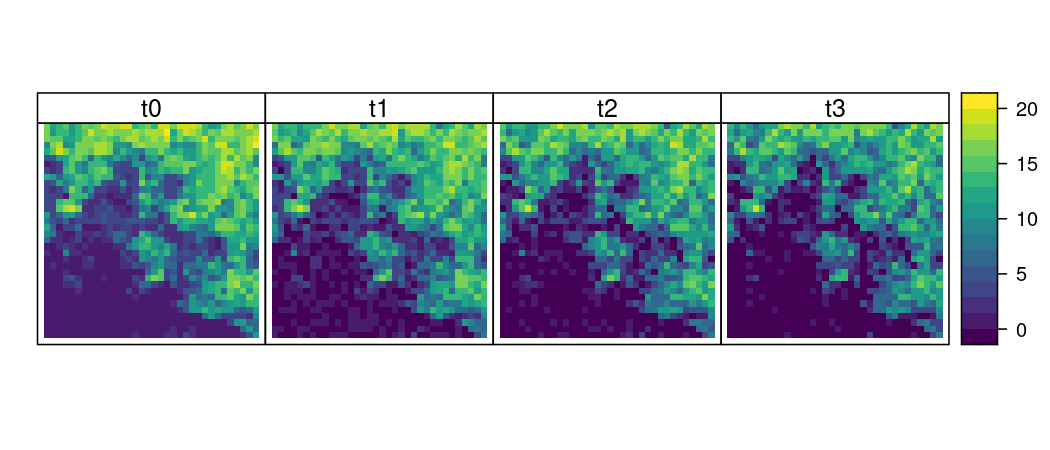Because of the internal organisation and structure of steps, we are also able to use any information contained within the landscape object in our custom functions. For example, say we would like to reduce the populations in only highly suitable habitat (above a threshold value) - this can be parameterised in the function.

We add an additional parameter to specify a threshold value indicating which cells in the landscape that we will randomly reduce populations in. To do this, we must also extract information from the suitability layer in the landscape object:

percent_mortality_hab <- function (percentage, threshold, stages = NULL) {

pop_dynamics <- function (landscape, timestep) {
population_raster <- landscape$population nstages <- raster::nlayers(population_raster) # get population as a matrix idx <- which(!is.na(raster::getValues(population_raster[]))) population_matrix <- raster::extract(population_raster, idx) # get suitability cell indexes suitable <- raster::extract(landscape$suitability, idx)

if (is.null(stages)) stages <- seq_len(nstages)
for (stage in stages) {
initial_pop <- sum(population_matrix[ , stage])
changing_pop <- sum(population_matrix[ , stage])

highly_suitable <- which(suitable >= threshold) # check for suitable cells

while (changing_pop > initial_pop * (1 - percentage)) {
non_zero <- which(population_matrix[ , stage] > 0)
i <- sample(intersect(highly_suitable, non_zero), 1) # change the sampling pool
population_matrix[i , stage] <- population_matrix[i , stage] - 1
changing_pop <- sum(population_matrix[ , stage])
}
}

population_raster[idx] <- population_matrix
landscape$population <- population_raster landscape } }  Now we run our simulation again using the new custom function and a suitability threshold of 0.7. Note, we must also now include a suitability raster layer in the landscape object or else our function will not work: ls <- landscape(population = egk_pop, suitability = egk_hab, carrying_capacity = NULL) pd <- population_dynamics(change = NULL, dispersal = NULL, modification = percent_mortality_hab(percentage = .10, threshold = 0.7, stages = 1), density_dependence = NULL) results <- simulation(landscape = ls, population_dynamics = pd, habitat_dynamics = NULL, demo_stochasticity = "none", timesteps = 3, replicates = 1, verbose = FALSE) Again, we can view the initial total juvenile landscape population and the modified population in each of the timesteps: cbind(c("t0", "t1", "t2", "t3"), c(cellStats(egk_pop[], sum), cellStats(results[][][["population"]][], sum), cellStats(results[][][["population"]][], sum), cellStats(results[][][["population"]][], sum))) ## [,1] [,2] ## [1,] "t0" "9066" ## [2,] "t1" "8159" ## [3,] "t2" "7343" ## [4,] "t3" "6608" And here is an updated plot of the juvenile cell populations: pop_stack <- stack(egk_pop[], results[][][["population"]][], results[][][["population"]][], results[][][["population"]][]) names(pop_stack) <- c("t0", "t1", "t2", "t3") par(mar=c(0,0,0,0), oma=c(0,0,0,0)) spplot(pop_stack, col.regions = viridis(100), par.settings=list(strip.background = list(col = "white"))) ## Warning in wkt(obj): CRS object has no comment ## Warning in wkt(obj): CRS object has no comment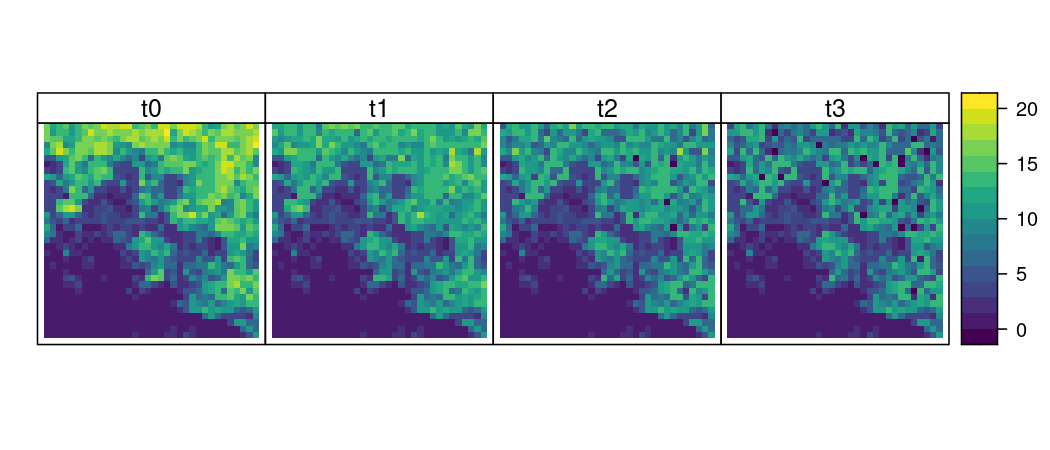Notice that whilst the total juvenile population numbers have decreased in a similar fashion, the spatial locations of the reductions have been confined to areas where habitat is highly suitable - as specified by our threshold value. But what if we want to influence the locations of the random samples by our own custom raster layer? This is accomplished by creating/loading a new raster that matches the properties (extent, resolution, and projection) of the population raster stack and adding it to the landscape object: sampling_mask <- egk_hab # copy the habitat layer to assume its properties sampling_mask[!is.na(egk_hab)] <- 0 # set all non-NA values to zero # get index values of the top-right corner (15 x 15 cells) idx_corner <- sort(unlist(lapply(0:15, function (x) seq(21, 541, by = 36) + x))) # set non-NA raster values in top-right corner to one sampling_mask[intersect(idx_corner, which(!is.na(egk_hab[])))] <- 1 plot(sampling_mask, axes = FALSE, box = FALSE)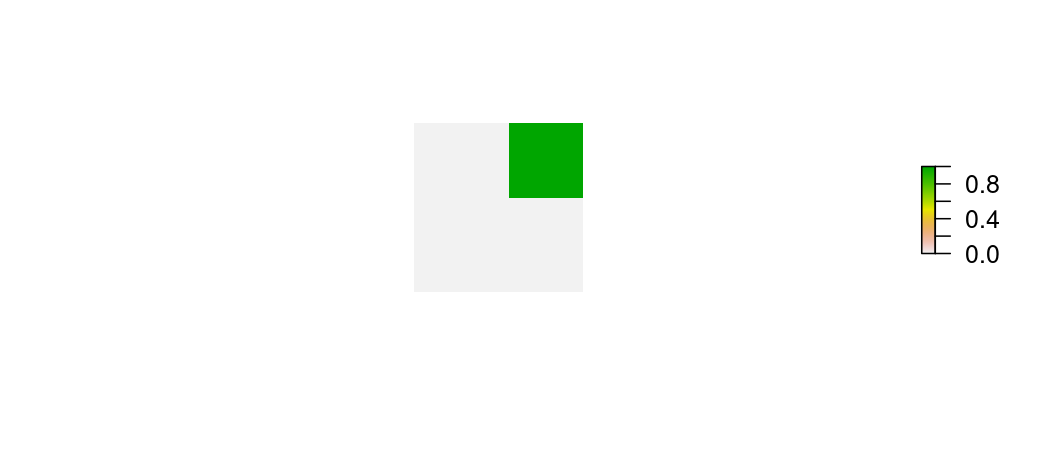ls <- landscape(population = egk_pop, suitability = NULL, carrying_capacity = NULL, "sampling_mask" = sampling_mask) # name the object to reference in our function We now need to slightly alter our custom function to accept and use the new raster. We start with the same syntax, adding a parameter to specify the name of the raster in the landscape object to use in our function and then extracting using the specified name: percent_mortality_ras <- function (percentage, masking_raster, threshold, stages = NULL) { pop_dynamics <- function (landscape, timestep) { population_raster <- landscape$population
nstages <- raster::nlayers(population_raster)

# get population as a matrix
idx <- which(!is.na(raster::getValues(population_raster[])))
population_matrix <- raster::extract(population_raster, idx)

# Note, here we now use the name of the raster ('masking_raster' parameter)
# to extract the object from the landscape object

if (is.null(stages)) stages <- seq_len(nstages)
for (stage in stages) {
initial_pop <- sum(population_matrix[ , stage])
changing_pop <- sum(population_matrix[ , stage])

highly_suitable <- which(suitable >= threshold) # check for suitable cells

while (changing_pop > initial_pop * (1 - percentage)) {
non_zero <- which(population_matrix[ , stage] > 0)
i <- sample(intersect(highly_suitable, non_zero), 1) # change the sampling pool
population_matrix[i , stage] <- population_matrix[i , stage] - 1
changing_pop <- sum(population_matrix[ , stage])
}
}

population_raster[idx] <- population_matrix
landscape$population <- population_raster landscape } }  In the ‘population_dynamics’ function, we change the modification parameter to call the new custom function (percent_mortality_ras) and add the new parameter specifying the name of the raster in the landscape object. Since we used zeros and ones in our sampling mask it does not matter which value we select for the threshold - as long as it is between zero and one. As previously, we run the simulation for a single replicate of three iterations: pd <- population_dynamics(change = NULL, dispersal = NULL, modification = percent_mortality_ras(percentage = .10, masking_raster = "sampling_mask", threshold = 0.7, stages = 1), density_dependence = NULL) results <- simulation(landscape = ls, population_dynamics = pd, habitat_dynamics = NULL, demo_stochasticity = "none", timesteps = 3, replicates = 1, verbose = FALSE) The changes in total juvenile landscape population are as expected: cbind(c("t0", "t1", "t2", "t3"), c(cellStats(egk_pop[], sum), cellStats(results[][][["population"]][], sum), cellStats(results[][][["population"]][], sum), cellStats(results[][][["population"]][], sum))) ## [,1] [,2] ## [1,] "t0" "9066" ## [2,] "t1" "8159" ## [3,] "t2" "7343" ## [4,] "t3" "6608" But now the changes in juvenile cell populations are confined to our ‘sampling_mask’ layer: pop_stack <- stack(egk_pop[], results[][][["population"]][], results[][][["population"]][], results[][][["population"]][]) names(pop_stack) <- c("t0", "t1", "t2", "t3") par(mar=c(0,0,0,0), oma=c(0,0,0,0)) spplot(pop_stack, col.regions = viridis(100), par.settings=list(strip.background = list(col = "white"))) ## Warning in wkt(obj): CRS object has no comment ## Warning in wkt(obj): CRS object has no comment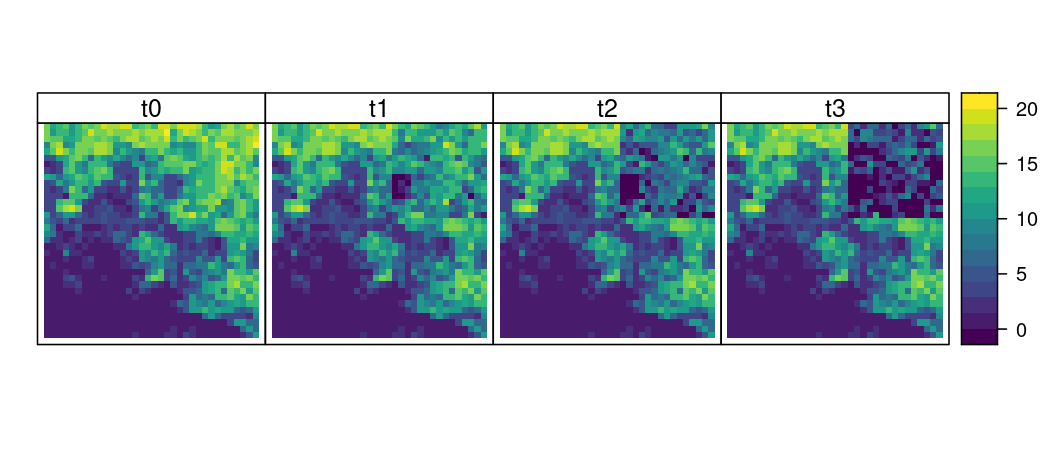So far we have been modifying the population rasters in the landscape object, but we can also modify vital rates as well. This may be useful in situations where a user would like to deterministically define, or affect, survival and fecundity. The ‘growth’ population dynamics function in steps includes a parameter for specifying a custom function (transition_function) to modify vital rates throughout a simulation. To start, let’s examine a pre-existing transition function called ‘modified_transition’ which affects survival and fecundity based on raster values: modified_transition <- function(survival_layer = NULL, fecundity_layer = NULL) { fun <- function (transition_array, landscape, timestep) { transition_matrix <- transition_array[, , 1] idx <- which(transition_matrix != 0) is_recruitment <- upper.tri(transition_matrix)[idx] array_length <- dim(transition_array) cell_idx <- which(!is.na(raster::getValues(landscape$population[])))

if (is.null(survival_layer)) {
surv_mult <- rep(1, length(cell_idx))
} else {
if (raster::nlayers(landscape$suitability) > 1) { surv_mult <- landscape[[survival_layer]][[timestep]][cell_idx] } else { surv_mult <- landscape[[survival_layer]][cell_idx] } } if (is.null(fecundity_layer)) { fec_mult <- rep(1, length(cell_idx)) } else { if (raster::nlayers(landscape$suitability) > 1) {
fec_mult <- landscape[[fecundity_layer]][[timestep]][cell_idx]
} else {
fec_mult <- landscape[[fecundity_layer]][cell_idx]
}
}

for (i in seq_len(array_length)) {
transition_array[, , i][idx[!is_recruitment]] <- transition_array[, , i][idx[!is_recruitment]] * surv_mult[i]
transition_array[, , i][idx[is_recruitment]] <- transition_array[, , i][idx[is_recruitment]] * fec_mult[i]
}

transition_array

}

as.transition_function(fun)

}

As with our original pre-built function “mortality”, the first statement names the function object and defines any global parameters that will be passed to the function - in this case raster objects specified by the user. The next statement defines an internal function (returned by the main function) and this is where the dynamic operations are specified. Note the two familiar parameters “landscape” and “timestep” and a third additional parameter “transition_array” - these are all required. The new parameter ‘transition_array’ is required since this function operates within the ‘growth’ function where a transition array is constructed from the ‘transition_matrix’ parameter and then passed around and operated on internally. The z-dimension of the transition array matches the number of cells in the landscape.

The next few statements determine which indices in the transition matrix refer to survival and fecundity and are non-zero (i.e. specified), and identify non-NA cells in the population rasters. The non-NA cells indices are used to exclude transition matrices that correspond to cells that cannot contain populations.

The function then extracts the values of survival and fecundity from the input rasters based on the recorded indices - first survival, then fecundity. If a raster layer is specified, its values are extracted - note, the code is written to accommodate both single rasters and raster stacks where the number of layers matches the intended timesteps of a simulation. If a raster layer is not specified, values of ones are stored.

In the next few statements of the internal function (‘fun’), the transition values are multiplied by the extracted values of the rasters (or ones). Similar to our original ‘mortality’ function example, the last statement in the internal function is always “transition_array” so the transition array object is returned when the internal function concludes its operations. The final statement assigns a class to the function - once again, this is optional but useful to maintain an organised structure in the software.

In the next example, we demonstrate the use of rasters to define the fecundity of adult kangaroos. We begin with a simple simulation of population growth. For this simulation we only need initial populations and a growth function based on a transition matrix - environmental stochasticity is set to zero by default in the function. We run the simulation for a single replicate of five iterations:

ls <- landscape(population = egk_pop,
suitability = NULL,
carrying_capacity = NULL)

pd <- population_dynamics(change = growth(transition_matrix = egk_mat),
dispersal = NULL,
modification = NULL,
density_dependence = NULL)

results <- simulation(landscape = ls,
population_dynamics = pd,
habitat_dynamics = NULL,
timesteps = 5,
replicates = 1,
verbose = FALSE)

Let’s look at how the total juvenile population changes through time:

plot_pop_trend(results, stages = 1)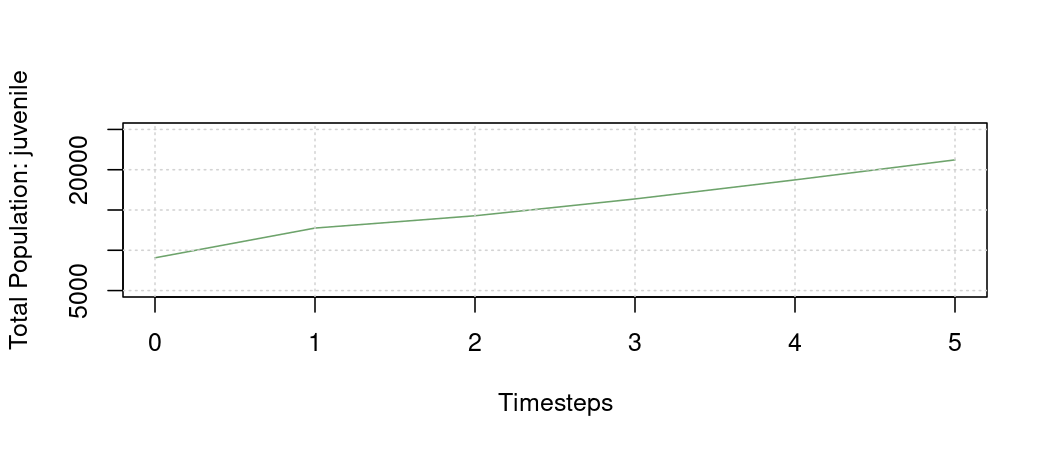And here is the spatial distribution of cell populations for juvenile kangaroos through time:

plot_pop_spatial(results,
stage = 1,
timesteps = 1:5)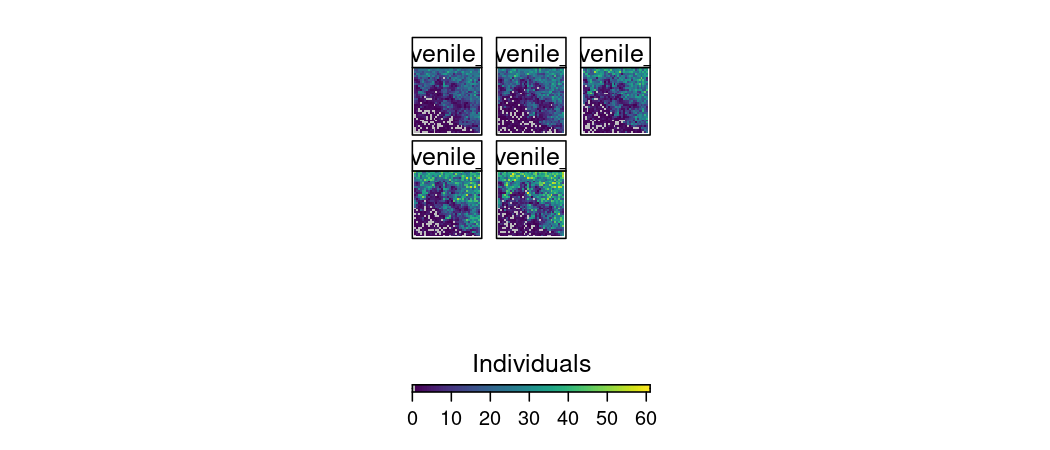If we look at the transition matrix used in the simulation, we can see why there is a steady increase in population (Rmax ~ 1.14):

##          juvenile subadult adult
## juvenile      0.0     0.55  0.85
## adult         0.0     0.55  0.85
##  "Rmax = 1.14194813396265"

Now let’s suppose that we want to provide rasters that define the fecundity of adult kangaroos. The methods are similar to our earlier examples but we will need to tailor our custom function to work within the pre-existing ‘growth’ dynamics function (type ?growth for more information). First, we will construct a raster that will contain our values of influence - in this case numbers that define adult fecundity in each cell (missing values will use existing fecundity). We do this randomly as an example, but users can read in spatial-explicit data that represents mapped factors affecting fecundity/survival (e.g. additional food/water, protection from predators, disease status):

adult_fec <- egk_hab # copy the habitat layer to assume its properties
adult_fec[] <- NA # set all values to NA

# assign random numbers between 0.1 and 0.5 to 80% of the cells
adult_fec[sample(seq_len(ncells), 0.8 * ncells, replace = FALSE)] <- runif(ncells, 0.1, 0.5)

plot(adult_fec, axes = FALSE, box = FALSE)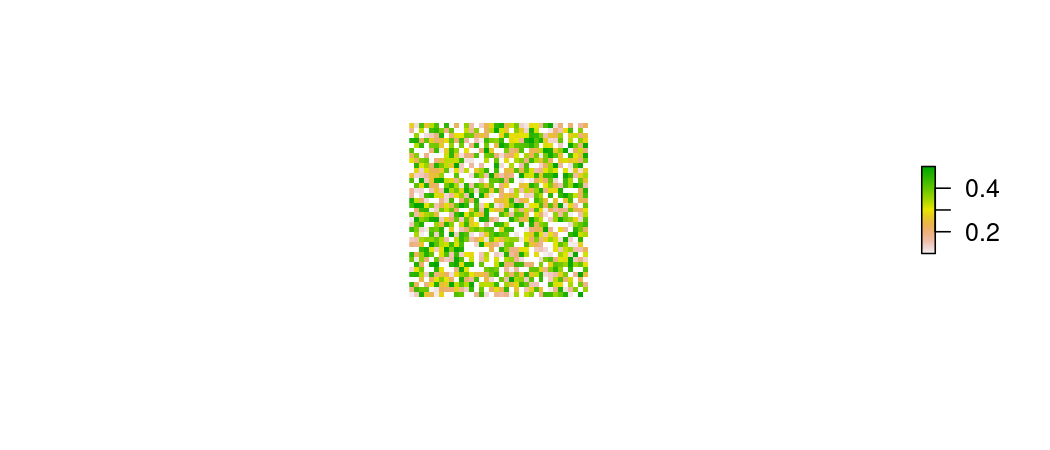Now let’s go about creating our custom function to define the fecundity of adult kangaroos. We start with the first two lines coded similar to the previous ‘modified_transition’ example and change the function name and retain only the fecundity_layer parameter:

define_fecundity <- function(fecundity_layer) {

fun <- function (transition_array, landscape, timestep) { ...

Next we define all of the indexing required to move values from the raster to the transition array (excluding zeros in the transition array and NA cells in the population raster):

define_fecundity <- function(fecundity_layer) {

fun <- function (transition_array, landscape, timestep) {
transition_matrix <- transition_array[, , 1]
idx <- which(transition_matrix != 0)
is_recruitment <- upper.tri(transition_matrix)[idx]
cell_idx <- which(!is.na(raster::getValues(landscape$population[]) # this length should be pre-defined by non-NA cells in the landscape array_length <- dim(transition_array) ... We then extract the new fecundity values from the user specified raster in the landscape object and assign them to their respective positions in the transition array: define_fecundity <- function(fecundity_layer) { fun <- function (transition_array, landscape, timestep) { transition_matrix <- transition_array[, , 1] idx <- which(transition_matrix != 0) is_recruitment <- upper.tri(transition_matrix)[idx] cell_idx <- which(!is.na(raster::getValues(landscape$population[])

# this length should be pre-defined by non-NA cells in the landscape
array_length <- dim(transition_array)

fec_values <- landscape[[fecundity_layer]][cell_idx]

for (i in seq_len(array_length)) {
new_fec <- fec_values[i]
if (!is.na(new_fec)) {
transition_array[, , i][tail(idx[is_recruitment], 1)] <- new_fec
}
} ...

Finally, similar to the original example, we return the modified transition array from the function:

define_fecundity <- function(fecundity_layer) {

fun <- function (transition_array, landscape, timestep) {
transition_matrix <- transition_array[, , 1]
idx <- which(transition_matrix != 0)
is_recruitment <- upper.tri(transition_matrix)[idx]
cell_idx <- which(!is.na(raster::getValues(landscape\$population[])))

# this length should be pre-defined by non-NA cells in the landscape
array_length <- dim(transition_array)

fec_values <- landscape[[fecundity_layer]][cell_idx]

for (i in seq_len(array_length)) {
new_fec <- fec_values[i]
if (!is.na(new_fec)) {
transition_array[, , i][tail(idx[is_recruitment], 1)] <- new_fec
}
}

transition_array
}
}

In the ‘population_dynamics’ function, we specify ‘growth’ in the ‘change’ parameter, use the ‘transition_function’ parameter to call the new custom function (define_fecundity), and add the new parameter specifying the name of the raster in the landscape object. As previously, we run the simulation for a single replicate of five iterations:

ls <- landscape(population = egk_pop,
suitability = NULL,
carrying_capacity = NULL,

pd <- population_dynamics(
change = growth(transition_matrix = egk_mat,
dispersal = NULL,
modification = NULL,
density_dependence = NULL)

results <- simulation(landscape = ls,
population_dynamics = pd,
habitat_dynamics = NULL,
timesteps = 5,
replicates = 1,
verbose = FALSE)

Let’s look at how the total juvenile population now changes through time (much slower due to the reduced fecundity of adults):

plot_pop_trend(results, stages = 1)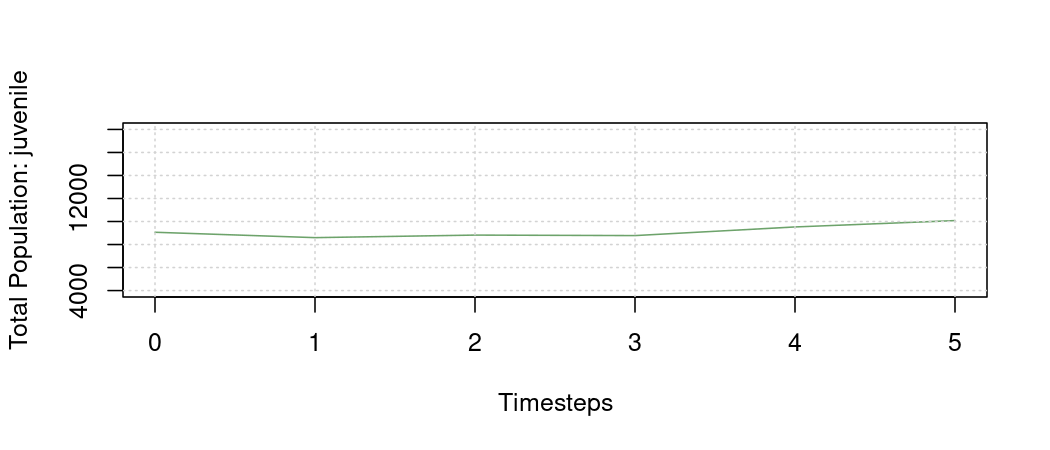And here is the updated spatial distribution of cell populations for juvenile kangaroos through time:

plot_pop_spatial(results,
stage = 1,
timesteps = 1:5)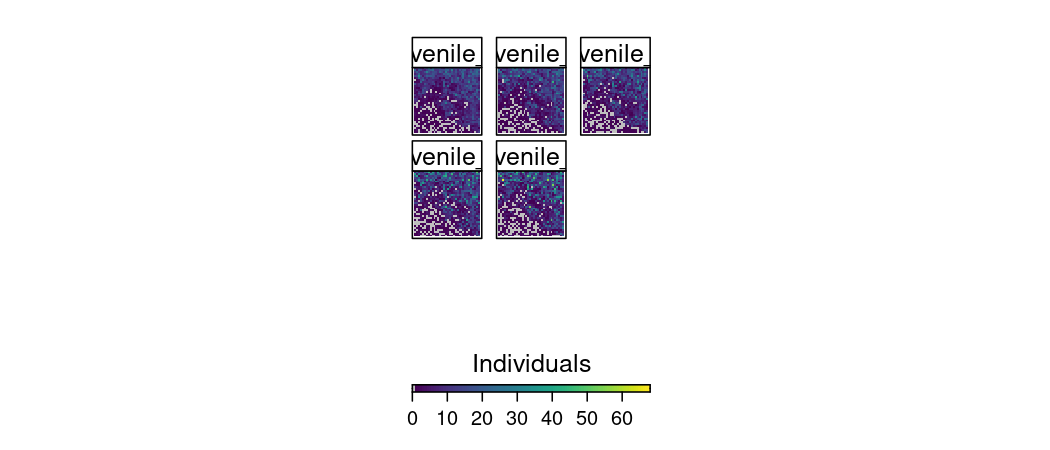Often analysts would like to specify custom dispersal kernels. As with the other customisations, the dispersal kernel requires a particular structure of nested functions. Let’s begin by reviewing an existing dispersal kernel function:

exponential_dispersal_kernel <- function (distance_decay = 0.5, normalize = FALSE) {
if (normalize) {
fun <- function (r) (1 / (2 * pi * distance_decay ^ 2)) * exp(-r / distance_decay)
} else {
fun <- function (r) exp(-r / distance_decay)
}
as.dispersal_function(fun)
}
r <- seq(1, 1000, 10)
distance_decay = 100
plot(r, exp(-r / distance_decay), type = 'l')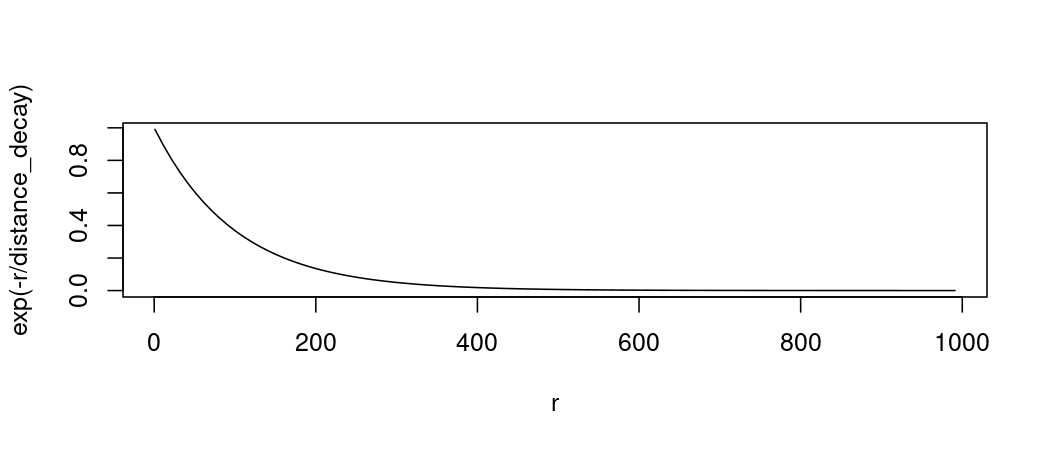We start with a wrapper function that accepts any general parameters - these are optional, of course. Examples may be parameters that control the shape of a curve that relates distance to proportion of dispersing individuals. The next few lines define two alternative dispersal kernel equations (a normalised variant of the other) which are selected by a parameter. Note, the internal functions must take a single parameter - in this case “r”, which is distance. The last line assigns a class to the object and is not required for customisation. So let’s create a simple logistic function for dispersal:

logistic_dispersal_kernel <- function (dist_shape, dist_slope) {
fun <- function (dist) 1 / (1 + exp((dist - dist_shape) / dist_slope))
fun
}

r <- seq(1, 1000, 10)
dist_shape = 500
dist_slope = 20
plot(r, 1 / (1 + exp((r - dist_shape) / dist_slope)), type = 'l')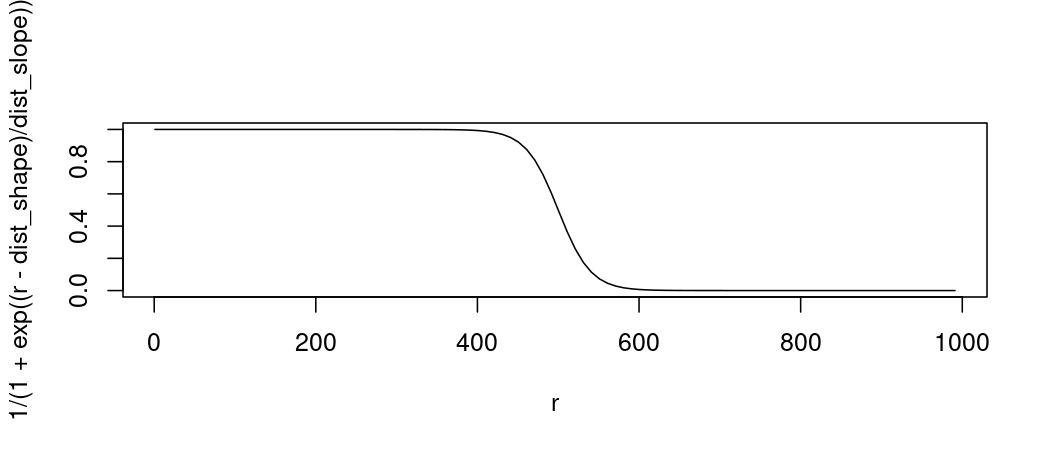Note, that the wrapper structure is still retained and that this function must return a nested function (called “fun” on the last line). The nested function takes in one parameter (dist) which can either be a single number or a vector of numbers - be sure your function can accommodate both.

Now, let’s test our dispersal function and compare it to the default exponential dispersal kernel. We will first thin out an example initial population raster stack so we can better see the effect of the new dispersal function.

egk_pop_thin <- egk_pop
egk_pop_thin[sample(1:ncell(egk_pop), 0.99 * ncell(egk_pop))] <- 0

ls <- landscape(population = egk_pop_thin,
suitability = NULL,
carrying_capacity = NULL)

pd_exp_disp <- population_dynamics(
change = NULL,
dispersal = kernel_dispersal(dispersal_kernel = exponential_dispersal_kernel(distance_decay = 100),
max_distance = 1000,
arrival_probability = "none"),
modification = NULL,
density_dependence = NULL)

results_01 <- simulation(landscape = ls,
population_dynamics = pd_exp_disp,
habitat_dynamics = NULL,
timesteps = 10,
replicates = 1,
verbose = FALSE)
##  "Kernel-based dispersal utilising available RAM to speed up operations"
plot_pop_spatial(results_01, stage = 3, timesteps = c(1, 5, 10))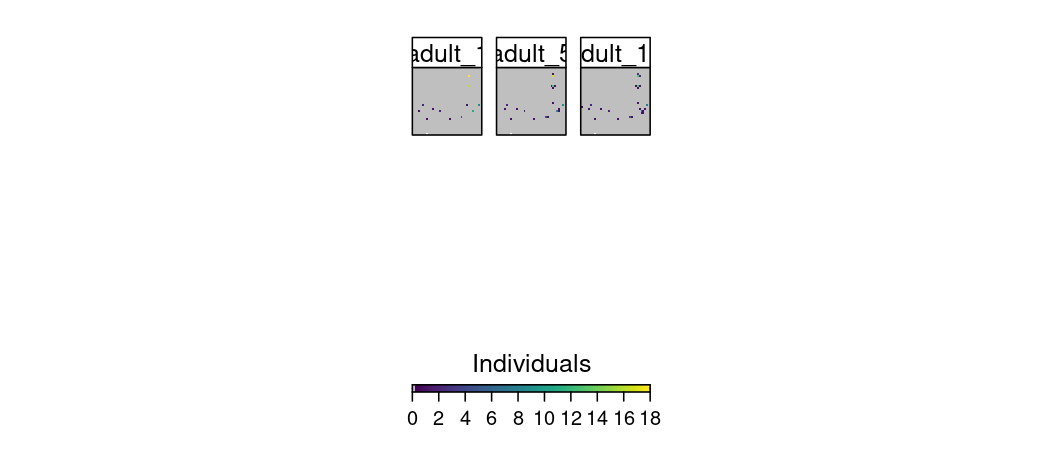pd_log_disp <- population_dynamics(
change = NULL,
dispersal = kernel_dispersal(dispersal_kernel = logistic_dispersal_kernel(dist_shape = 500, dist_slope = 20),
max_distance = 1000,
arrival_probability = "none"),
modification = NULL,
density_dependence = NULL)

results_02 <- simulation(landscape = ls,
population_dynamics = pd_log_disp,
habitat_dynamics = NULL,
timesteps = 10,
replicates = 1,
verbose = FALSE)
##  "Kernel-based dispersal utilising available RAM to speed up operations"
plot_pop_spatial(results_02, stage = 3, timesteps = c(1, 5, 10))
## Warning in graphics::par(op): calling par(new=TRUE) with no plot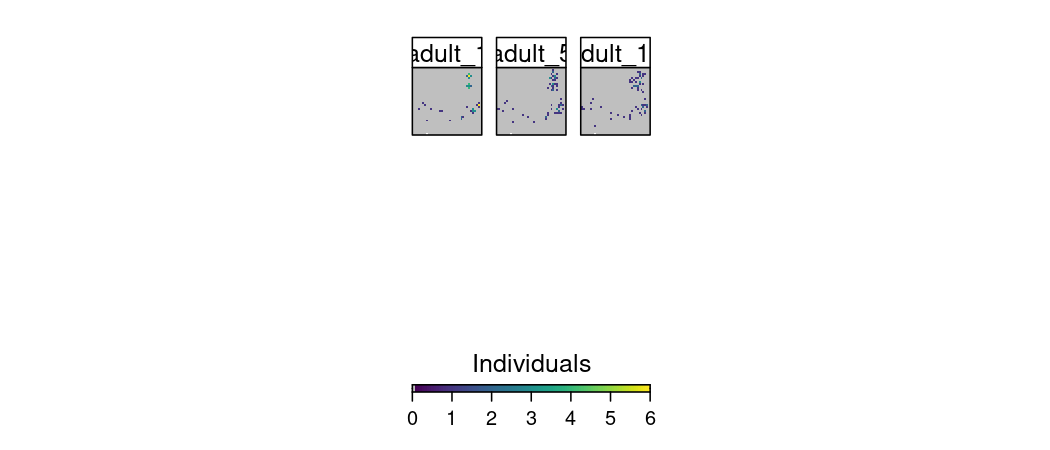Please note that if any custom functions are used in a simulation that is run in parallel (e.g. plan(multisession)), then a list of the functions and any related objects must be passed into the simulation function using the future.globals argument:

plan(multisession)
results_02 <- simulation(landscape = ls,
population_dynamics = pd_log_disp,
habitat_dynamics = NULL,
timesteps = 10,
replicates = 3,
verbose = FALSE,
future.globals = list("logistic_dispersal_kernel" = logistic_dispersal_kernel))

These are simple examples, but users can specify a seemingly endless configuration of dynamics to be used throughout a simulation by following the structures and methods introduced in this vignette. Anything can be stored in a landscape object and then be referred to within a custom function. Thus, the procedure for creating custom functions also applies to habitat dynamics - which are called in the simulation function.

We encourage users to examine the pre-built functions in steps to get an idea of their internal operations. Further, these pre-built functions can serve as templates to get users started in customising and creating their own functions.